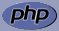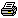www.PHPBuddy.comPHP Function Lookup:

 Categories
 PHP Quick Start PHP Installation PHP Articles PHP Scripts

 Top Rated Articles

 Site Related
 Submit Articles/Code Contact UsArticle Added on: April 16, 2002
Basic LoopingOverview: Understanding how to use looping in our code introduction to While Loops

The ability to add logic in a script is the first fundamental part of any true programming language, but then the ability to execute the same code multiple times is also very important.

Imagine you had to print the number from 1 to 20 you could echo it from 1....20 or we can write a simple while loop that does the job.

Introducing the While Loop
while loops are the simplest type of loop in PHP, It tells PHP to execute the nested statement(s) repeatedly, as long as the while expression evaluates to TRUE.

The syntax of a while loop ``` While(conditions) { // This code will execute until the conditions // provided no longer evaluates to true }```

Let do a simple while loop that prints the numbers 1 to 10

```<?php \$cnt = 1; while(\$cnt != 11) { echo "\$cnt "; \$cnt = \$cnt + 1; } ?>```

Understanding the above example: In the above example we have created a variable \$cnt and assigned it an value of 1, then we add the while loop.

We said to the while loop to continue the loop until \$cnt != 11 means that the loop should countinue until \$cnt reaches 11, then we print the value of \$cnt, after that we increment the value of \$cnt by one, the loop checks the value of \$cnt if its not equal to 11 it continues the loop, when finally \$cnt reaches 11 the loop exits.

Let's make a simple multiplication table using While Loop

```<?php \$table = 5; //we will use the multiplication table 5 \$cnt = 1; while(\$cnt != 11) { echo " \$table x \$cnt = ". \$table * \$cnt; \$cnt++; } ?>```

See how easy it is to use while loops!Always be sure to incriment the counter and check the logic in the while loop so that it exit's upon reaching certain condition or value otherwise you might end up with a infinte loop (A loop that goes on and on and never exits).